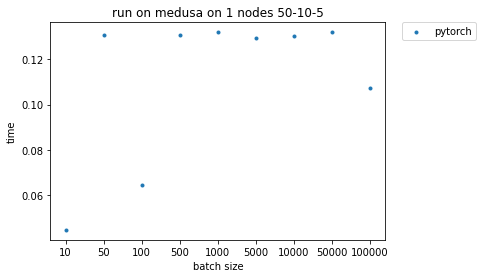# Data parallelization in PyTorch

I’m running a simple code performing 1D convolution on an input array, and a given filter specification, as follows:

``````import argparse
import sys
import torch.nn as nn
import torch
import time

parser = argparse.ArgumentParser(description='Parameters')
help='batch, length, out_channels, kernel_size')

args = parser.parse_args()

batch = args.integers
length = args.integers
out_channels = args.integers
kernel_size = args.integers

array = torch.rand(batch, 3, length)
conv1 = nn.Conv1d(in_channels=3,out_channels=out_channels, kernel_size=kernel_size, bias=False)

t_start = time.time()
out = conv1(array)
t_end = time.time()
t = t_end-t_start

``````

I run my code on a single machine with 40 cores. When I measure the time I see that for some filter lengths I don’t see any performance degradation when the number of batches increases, which is strange. Since I’m not even using `nn.parallel` or `distributed` module, so my question is does these results make sense? How is PyTorch designed to handle data parallelization in this case? Is there a threshold for batch size from which it starts parallelization? If it does parallelization does it simply divide the batches among the cores and assigns each chunk to a core?
The following image shows the results of running the above-mentioned code on one node while keeping input length, filter length, and out channels fixed (50, 10 ,5 in this image) and increasing the batch size.You typically have two forms of parallelism on the CPU, intra-op (so one operation is executed on several CPUs) and inter-op (so several operations are launched in parallel).
You might see that the first works better for larger batches.